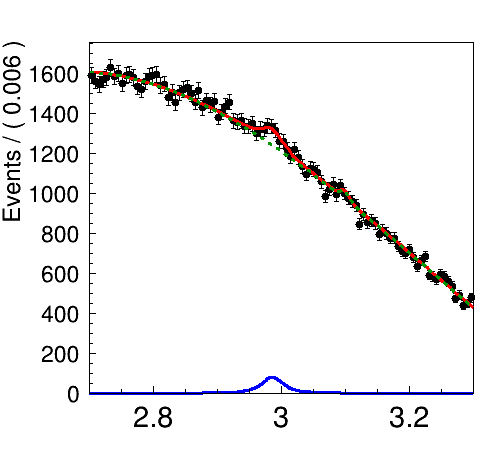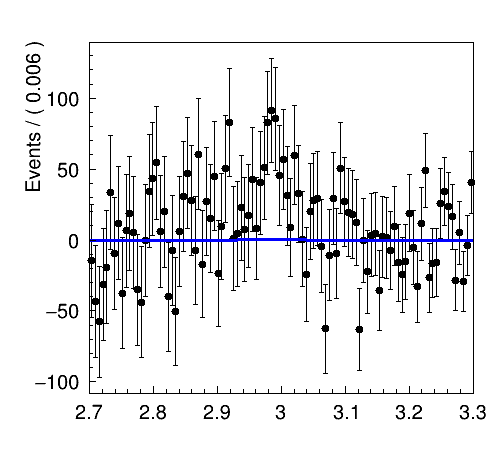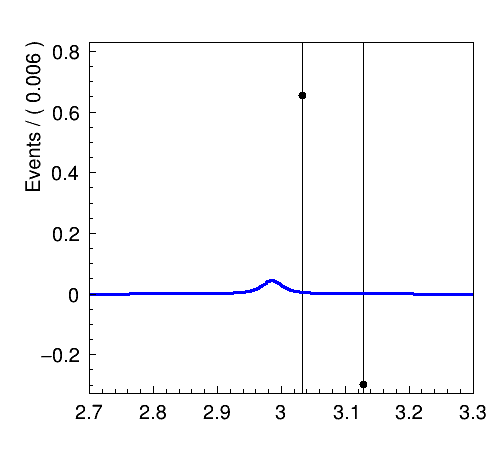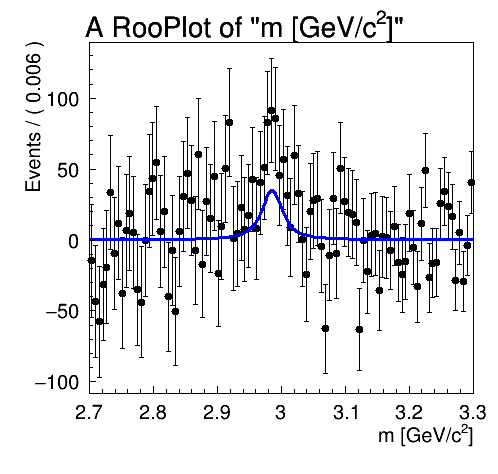# RooSimultaneous plot components on subtracted data spectrum

Hi,

I have a problem to plot part of a RooSimultaneous on a background subtracted spectrum, the normalization seems to be completely off.

What I did:

a) Fit a RooSimultaneous to a combined RooDataHist (16 datasets in total); fit model consists of a RooVoigtian as signal an RooChebychev as background.

b) I plotted the result projected to one spectrum with (I skipped line style options in the code snippets):

``````  RooPlot *framecomb = x->frame();

// this is the combined dataset
combData.plotOn(framecomb);

// simPdf is the RooSimultaneous
simPdf.plotOn(framecomb, ProjWData(combData), LineColor(kRed));

// comps is a TString holding the 16 names of the background functions, compssig the names of the signal components
simPdf.plotOn(framecomb, ProjWData(combData), Components(comps.Data()), LineColor(kGreen+2));
simPdf.plotOn(framecomb, ProjWData(combData), Components(compssig.Data()), LineColor(kBlue));
``````

The result looks like this:What I want to do:
a) Create and plot a background subtracted spectrum, where the green background is subtracted from the combined RooDataHist combData. I didn’t find any simple solution, so I made some brute-force approach to create the subtracted spectrum:

``````TH1 *htot = combData.createHistogram("htot",*x,Binning(100,xmin,xmax));
TH1F *hbkg = new TH1F("hbkg","",100,xmin,xmax);

for (int i=1;i<=16;++i)
{
RooRealVar *b = w->var(Form("B%d",i));  // this is the number of background events, w is a RooWorkspace
TH1* htmp = w->pdf(Form("cpol%d",i))->createHistogram("hbkg",*x,Binning(100,xmin,xmax)); //cpol_i are the Chebychev polynomials
htmp->Scale(b->getVal());
delete htmp;
}

for (int i=0;i<=htot->GetNbinsX(); ++i)
htot->SetBinContent(i, htot->GetBinContent(i) - hbkg->GetBinContent(i));

RooDataHist *hsub = new RooDataHist("hsub","",*x, Import(*htot));
``````

b) Plot on top the blue combined signal pdf.

What I tried so far is:

``````RooPlot *framesub = x->frame("Subtracted spectrum");
hsub->plotOn(framesub);
simPdf.plotOn(framesub, ProjWData(combData),  Components(compssig.Data()), LineColor(kBlue));
framesub->Draw();
``````

The outcome isIt looks like if the blue line is flat, but when you zoom in you see that it is the right pdf, but normalized wrongly:I already tried to compensate this with the option Normalization(sum_events, RooAbsReal::Relative), which scales it up but still doesn’t match the real line shape.Can anybody help me with that?

Best regards and thanks,
Klaus

@StephanH can you help here?

Hello Klaus,

PDFs are automatically normalised to the amount of data in the plot. If you subtract, that amount may be wrong.
I would advise to use an explicit normalisation (`RooAbsReal::NumEvent` instead of relative) for the background-subtracted plot to force the signal to the same number of events as in the non-subtracted plot.

There’s two options:

• You plot the full model, but select only the signal component. In that case, use the total number of events in the non-subtracted plot (RooDataHist::sumEntries())
• You plot the signal model only. In that case, you need to compute the number of events of the signal component in the non-subtracted plot. That’s certainly possible, but involves computing the signal fraction in the original plot.

Hi Stephan,

I think the problem in this “special” case is, that the plot comes from a simultaneous fit, which is create somehow (I don’t really understand how it works) with the option ProjWData(combData). For me it seems that there is the issue.

I followed both of your recommendations, the results are attached

left) `simPdfSig.plotOn(frame1, ProjWData(combData), Normalization(sumev,RooAbsReal::NumEvent))`

where sumev is the fraction of the signal in the combined plot above (N = 786), simPdfSig is the combination of all signal pdfs

center) `simPdf.plotOn(frame2, ProjWData(combData), Normalization(combData.sumEntries(),RooAbsReal::NumEvent), Components(compssig.Data()))`

plot the components, scaled to the total number of events in the non-subtracted plot

right) `simPdf.plotOn(framesub3, ProjWData(*hsub), Normalization(sumev,RooAbsReal::NumEvent), Components(compssig.Data()))`
this was the try to use the subtracted RooDataHist hsub in the ProjWData options, which didn’t show at all any curve.

It seems that the pdfs are intransparently connected to the dataset they were fit to.

Is there some bin-width involved in the drawing of the pdf, which is screwed and leads to the wrong scaling of the pdf? I’m also puzzled about to what area this pdf is normalized at all. Is there a way to get the normalization for a specific RooPlot it is drawn on?

Best,
Klaus

Hello,

Indeed, the projection of the simultaneous on a background-subtracted plot is probably not straight forward. The reason you have to use ProjWData is exlained in this tutorial:
https://root.cern.ch/doc/master/rf501__simultaneouspdf_8C.html
A simultaneous PDF cannot be integrated over the category dimension, so you have to remove it by choosing a category.

Could it be that you have to slice the dataset, in order to only use the relevant category? At least that’s what’s done in the tutorial.

• The number of events of the last-plotted dataset is retrieved using `RooPlot::getFitRangeNEvt()`.